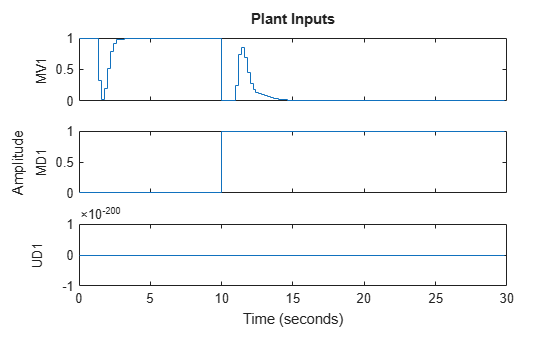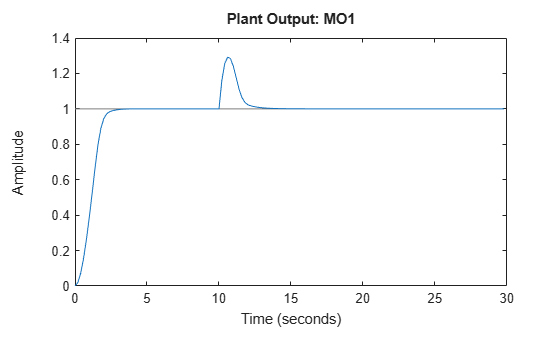Main Content

# sim

Simulate an MPC controller in closed loop with a linear plant

## Syntax

``sim(mpcobj,Ns,r)``
``sim(mpcobj,Ns,r,v)``
``sim(___,SimOptions)``
``[y,t,u,xp,xc,SimOptions] = sim(___)``

## Description

Use the Model Predictive Control Toolbox™ `sim` function to simulate the closed-loop or open-loop response of an MPC controller with constraints and weights that do not change at run time. The MPC controller can be implicit or explicit, the controlled plant must be linear and time-invariant, and you must specify the reference and disturbance signals in advance. By default, the plant used in the simulation is the one in `MPCobj.Model.Plant`, but you can use a different plant model to assess the controller robustness to model mismatch.

To run simulink models programmatically instead, see `sim` (Simulink).

````sim(mpcobj,Ns,r)` simulates the closed-loop system formed by the plant model specified in `mpcobj.Model.Plant` and by the MPC controller `mpcobj`, in response to the specified reference signal, `r`. The MPC controller can be either a traditional MPC controller (`mpc`) or explicit MPC controller (`explicitMPC`). The simulation runs for the specified number of simulation steps, `Ns`. `sim` plots the simulation results.```

example

````sim(mpcobj,Ns,r,v)` also specifies the measured disturbance signal `v`. ```
````sim(___,SimOptions)` specifies additional simulation options. This syntax allows you to alter the default simulation options, such as initial states, input/output noise, and unmeasured disturbances, plant mismatch, etc. It also allows you to simulate the plant in open loop. You can use `SimOptions` with any of the previous input combinations.```
````[y,t,u,xp,xc,SimOptions] = sim(___)` suppresses plotting and instead returns: the sequence of plant outputs `y`,the time sequence `t` (equally spaced by `mpcobj.Ts`),the manipulated variables `u` generated by the MPC controller,the sequence `xp` of states of the model of the plant used for simulation,the sequence `xmpc` of states of the MPC controller (provided by the state observer),and the simulation options object, `SimOptions`. ```

## Examples

collapse all

Simulate the MPC control of a MISO system. The system has one manipulated variable, one measured disturbance, one unmeasured disturbance, and one output.

Create the continuous-time plant model. This plant will be used as the prediction model for the MPC controller.

`sys = ss(tf({1,1,1},{[1 .5 1],[1 1],[.7 .5 1]}));`

Discretize the plant model using a sampling time of 0.2 units.

```Ts = 0.2; sysd = c2d(sys,Ts);```

Specify the MPC signal type for the plant input signals.

`sysd = setmpcsignals(sysd,'MV',1,'MD',2,'UD',3);`

Create an MPC controller for the `sysd` plant model. Use default values for the weights and horizons.

`MPCobj = mpc(sysd);`
```-->The "PredictionHorizon" property of "mpc" object is empty. Trying PredictionHorizon = 10. -->The "ControlHorizon" property of the "mpc" object is empty. Assuming 2. -->The "Weights.ManipulatedVariables" property of "mpc" object is empty. Assuming default 0.00000. -->The "Weights.ManipulatedVariablesRate" property of "mpc" object is empty. Assuming default 0.10000. -->The "Weights.OutputVariables" property of "mpc" object is empty. Assuming default 1.00000. ```

Constrain the manipulated variable to the `[0 1]` range.

`MPCobj.MV = struct('Min',0,'Max',1);`

Specify the simulation stop time.

`Tstop = 30;`

Define the reference signal and the measured disturbance signal.

```num_sim_steps = round(Tstop/Ts); r = ones(num_sim_steps,1); v = [zeros(num_sim_steps/3,1); ones(2*num_sim_steps/3,1)];```

The reference signal, `r`, is a unit step. The measured disturbance signal, `v`, is a unit step, with a 10 unit delay.

Simulate the controller.

`sim(MPCobj,num_sim_steps,r,v)`
```-->The "Model.Disturbance" property of "mpc" object is empty: Assuming unmeasured input disturbance #3 is integrated white noise. Assuming no disturbance added to measured output channel #1. -->The "Model.Noise" property of the "mpc" object is empty. Assuming white noise on each measured output channel. ```## Input Arguments

collapse all

Model predictive controller, specified as one of the following:

Number of simulation steps, specified as a positive integer.

If you omit `Ns`, the default value is the number of rows of whichever of the following arrays has the largest number of rows:

• The input argument `r`

• The input argument `v`

• The `UnmeasuredDisturbance` property of `SimOptions`, if specified

• The `OutputNoise` property of `SimOptions`, if specified

Example: `100`

Reference signal, specified as an array. This array has `ny` columns, where `ny` is the number of plant outputs. `r` can have anywhere from 1 to `Ns` rows. If the number of rows is less than `Ns`, the missing rows are set equal to the last row.

Example: `ones(100,1)`

Measured disturbance signal, specified as an array. This array has `nv` columns, where `nv` is the number of measured input disturbances. `v` can have anywhere from 1 to `Ns` rows. If the number of rows is less than `Ns`, the missing rows are set equal to the last row.

Example: `[zeros(50,1);ones(50,1)]`

Simulation options, used to specify additional simulation options as well as noise and disturbance signals that feed into the plant but are unknown to the controller. You can also use this object to simulate the plant in open loop, or to specify a plant model to be used in simulation that is different from the one in `MPCobj.Model.Plant`, which allows you to assess the robustness of the control loop response to model mismatch.

For more information, see `mpcsimopt`.

## Output Arguments

collapse all

Sequence of plant outputs values, returned as a `Ns`-by-`Ny` array, where `Ns` is the number of simulation steps and `Ny` is the number of plant outputs. The values in `y` include neither additive output disturbances nor additive measurement noise (if any).

Time sequence, returned as a `Ns`-by-1 array, where `Ns` is the number of simulation steps. The values in `t` are equally spaced by `MPCobj.Ts`.

Sequence of manipulated variables values generated by the MPC controller, returned as a `Ns`-by-`Nu` array, where `Ns` is the number of simulation steps and `Nu` is the number of manipulated variables.

Sequence of plant model states values, returned as an `Ns`-by-`Nxp` array, where `Ns` is the number of simulation steps and `Nxp` is the number of states in the plant model. The plant model is either `MPCobj.Model` or `SimOptions.Model`, if the latter is specified.

Sequence of MPC controller states, returned as an `Ns`-by-1 structure array. Each entry in the structure array has the same fields as an `mpcstate` object. At each time step, the MPC controller obtains the manipulated variable by multiplying an estimate of the plant state by a gain matrix (computed by solving a constrained quadratic optimization problem). By default, the controller uses a linear Kalman filter to estimate the state of the discrete time plant, augmented by the disturbance and noise models. Therefore, the states of the controller are the states of this Kalman filter, which are in turn the estimates of the plant, disturbance, and noise model states at each time step.

Simulation options objects used for the simulation. This object can specify noise and disturbance signals that feed into the plant but are unknown to the controller. It can also specify if the simulated system is open loop or if the plant model used in the simulation is different from the one in `MPCobj.Model.Plant`.

For more information, see `mpcsimopt`.

## See Also

Introduced before R2006a

## Support

#### Implementing an Adaptive Cruise Controller with Simulink

Download technical paper